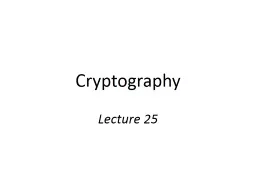# Cryptography Lecture 25 Public-key encryption - PowerPoint Presentation#### Cryptography Lecture 25 Public-key encryption - Description

pk sk pk c Enc pk m m Dec sk c c pk pk Publickey encryption A publickey encryption scheme is composed of three PPT algorithms Gen keygeneration algorithm ID: 706139 Download Presentation

### Tags

encryption key public ciphertext key encryption ciphertext public security cpa secure scheme private chosen attacks outputs message cca enc

Download Presentation - The PPT/PDF document "Cryptography Lecture 25 Public-key encry..." is the property of its rightful owner. Permission is granted to download and print the materials on this web site for personal, non-commercial use only, and to display it on your personal computer provided you do not modify the materials and that you retain all copyright notices contained in the materials. By downloading content from our website, you accept the terms of this agreement.

Embed / Share - Cryptography Lecture 25 Public-key encryption

## Presentation on theme: "Cryptography Lecture 25 Public-key encryption"— Presentation transcript

Slide1

Cryptography

Lecture 25Slide2

Public-key encryption

pk

,

sk

pk

c

Encpk(m)

m = Decsk(c)

c

pk

pkSlide3

Public-key encryption

A public-key encryption scheme is composed of three PPT algorithms:

Gen:

key-generation algorithm that on input 1n outputs pk,

skEnc: encryption algorithm that on input pk and a message m outputs a ciphertext c

Dec: decryption algorithm that on input sk and a ciphertext c outputs a message m or an error

3

For all

m and pk, sk output by Gen,

Decsk(Encpk(m)) = m

Slide4

CPA-security

Fix a public-key encryption scheme

Define experiment PubK-CPAA,

(n):Run Gen(1n) to get keys pk

, skGive pk to A, who outputs (m

0, m1) of same length

Choose uniform b  {0,1} and compute the ciphertext c  Encpk

(mb); give c to AA outputs a guess b’, and the experiment evaluates to 1 if b’=b4Slide5

CPA-security

Public-key encryption scheme

 is

CPA-secure if for all PPT adversaries A: Pr

[PubK-CPAA, (n

) = 1] ≤ ½ + negl(n)

5Slide6

Notes on the definition

No encryption oracle?!

Encryption oracle redundant in public-key setting

 No perfectly secret public-key encryption

No deterministic public-key encryption scheme can be CPA-secure CPA-security implies security for encrypting multiple messages as in the private-key case

6Slide7

Chosen-ciphertext

attacks

pk

,

sk

pk

c

 Enc

pk(m)c

c

m

’Slide8

Chosen-ciphertext attacks

Chosen-

ciphertext

attacks are arguably even a greater concern in the public-key settingAttacker might be a legitimate senderEasier for attacker to obtain full decryptions of

ciphertexts of its choice Related concern: malleabilityI.e., given a ciphertext c that is the encryption of an unknown message m, might be possible to produce

ciphertext c’ that decrypts to a related message m’This is also undesirable in the public-key setting

8Slide9

Chosen-ciphertext attacks

Can define CCA-security for public-key encryption by analogy to the definition for private-key encryption

See book for details

9Slide10

Hybrid encryption

10

k

pk

ciphertext

“encapsulated key”

The

functionality

of public-key encryption

at the (asymptotic)

efficiency

of private-key encryption!

Enc

Enc

m

Decryption done in the obvious waySlide11

Security of hybrid encryption

Let

 be the public-key component, and ’ the private-key component; let 

hy denote their combinationIf  is a CPA-secure public-key scheme, and ’ is a CPA-secure private-key scheme, then

hy is a CPA-secure public-key schemeSimilarly for CCA-security

11Slide12

For hybrid encryption, something

weaker

than public key encryption would sufficeSufficient to have an “encapsulation algorithm” that takes a public key and outputs a ciphertext/key pair (c, k)

Correctness: k is recoverable from c given skSecurity: k is indistinguishable from uniform given pk and cThis can lead to more-efficient constructionsSlide13

Dlog

-based PKESlide14

Diffie-Hellman key exchange

k = (h

2

)

x

m

= c

2

/k

k = (h1)

y

(G, q, g)  G(1n)

x  ℤq

h1 = g

x

G, q, g, h

1

y

q

h

2

=

g

y

h

2

c

2

= k · mSlide15

El Gamal encryption

k = (h

2

)

x

m

= c

2

/k

k = (h1

)y

(G, q, g)  G(1n)

x  ℤ

qh1 =

gx

G, q, g, h

1

y

q

h

2

=

g

y

h

2

c

2

= k · m

Public key

h

2

, h

1

y

· m Slide16

El Gamal encryption

Gen(1

n

)Run G(1

n) to obtain G, q, g. Choose uniform xℤq. The public key is (G, q, g,

gx) and the private key is xEncpk

(m), where pk = (G, q, g, h) and mG

Choose uniform y ℤq. The

ciphertext is gy, hy·mDec

sk(c1, c2)Output c2/c1

x

16Slide17

Security?

If the DDH assumption is hard for

G

, then the El Gamal encryption scheme is CPA-secureFollows from security of Diffie

-Hellman key exchange, or can be proved directly(Discrete-logarithm assumption alone is not enough here)17Slide18

In practice…

Parameters G, q, g are standardized and shared

Inconvenient to treat message as group element

Use key derivation to derive a key k instead, and use k to encrypt the messageI.e.,

ciphertext is gy, Enc’k(m),

where k = H(hy)Can be analyzed using KEM/DEM paradigm

18Slide19

Chosen-ciphertext attacks?

El

Gamal

encryption is not secure against chosen-ciphertext attacks

Follows from the fact that it is malleableGiven ciphertext c1, c2

, transform it to obtain the ciphertext c1, c’2 = c1

,  · c2 for arbitrary

Since c1, c2 = g

y, hy · m, we have c1, c’2 = gy

, hy · (m)I.e., encryption of m becomes an encryption of m!

19Slide20

Attack!

20

G, q, g, h

c

1

, c

2

c

1

, 2 ·c

2

(Assume 2

 G 

ℤ*p)

First bid: mSecond bid: 2mSlide21

Chosen-ciphertext security

Use key derivation coupled with CCA-secure private-key encryption scheme

I.e.,

ciphertext is

gy, Enc’k(m),where k = H(hy) and

Enc’ is a CCA-secure schemeCan be proved CCA-secure under appropriate assumptions, if H is modeled as a random oracleDHIES/ECIES

21

Shom More....